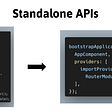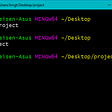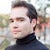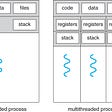# CoDEVIANT #19 — If there’s a problem yo I’ll solve itCheck out the hook while my DJ revolves it!

`function isMonotonic(array) {  let monoArr = []array.forEach((el, i) => {  if(el > array[i + 1] ) {   monoArr.push('negative')  } else if(el < array[i + 1]) {   monoArr.push('positive')  } })  monoArr = [... new Set(monoArr)]  if(monoArr.length == 1 || monoArr.length == 0) {  return true } else {  return false }}`
`let monoArr = []`
`array.forEach((el, i) => {  if(el > array[i + 1]) {    monoArr.push('negative')  } else if(el < array[i + 1]) {    monoArr.push('positive')  }})`
`monoArr = [... new Set(monoArr)]`
• I say that if monoArr.length equals zero or one, we return true
• I say that if monoArr.length is anything else, we return false
`if(monoArr.length == 1 || monoArr.length == 0) {  return true } else {  return false }`
`function isMonotonic(array) { if(array.length <= 2) {   return true } let direction = array - array for(let i = 2; i < array.length; i++){   if(direction === 0) {      direction = array[i] - array[i-1]      continue   }   if(breaksDirection(direction, array[i-1], array[i])){     return false   } }}function breaksDirection(direction, previousInt, currentInt) {  const difference = currentInt - previousInt  if(direction > 0) {    return difference < 0  }  return difference > 0}`
• We say up front that if the length of the array is 2 or less than two, we return true.
`if(array.length <= 2) {  return true}`
• We create a variable direction to equal the 2nd array minus the 1st array
`let direction = array - array`
• We create a for-loop and we start our counter variable, i at 2 so as to set our first value that we are comparing at array[i] to be the third element in our zero-indexed array. We keep the loop going so long as i is less than the length of the array and we increment i each rotation.
`for(let i = 2; i < array.length; i++ ){  ...}`
• Then we say that if direction equals 0. (i.e. the first two elements of the array are the same), then we set direction to instead equal the current value of the array in the for-loop **array[i]** minus the value before it **array[i -1]**. We also tell the computer to run the continue command. This, within the context of a for-loop, like what we’re in right now, skips the rest of the iteration and starts the next iteration of the for-loop. This is done so we can get to a point where direction does NOT equal zero.
`for(let i = 2; i < array.length; i++ ){  if(direction === 0) {    direction = array[i] - array[i - 1]    continue  }  ...}`
• Next, we create another if-statement inside our for-loop to see if a new method we’re about to create returns true and if so, to return false.
`for(let i = 2; i < array.length; i++ ){  if(direction === 0) {    direction = array[i] - array[i - 1]    continue  }  if(breaksDirection(direction, array[i-1], array[i])){    return false  }}`
• Let’s create our helper-method breaksDirection. Basically with the arguments passed in (direction, previousInt, currentInt), we create a variable called difference from subtracting previousInt from currentInt.
`function breaksDirection(direction, previousInt, currentInt) {  const difference = currentInt - previousInt}`
• We then say that if direction (an argument that was passed in) is more than 0, we need to return whether difference (the value we just calculated) is less than 0. If direction is less than 0, we need to return whether difference is more than 0.
`function breaksDirection(direction, previousInt, currentInt) {  const difference = currentInt - previousInt  if(direction > 0) {   return difference < 0  }  return difference > 0}`
• Back to the main function: Should the if-statement’s condition yield false for the rest of the for-loop iterations, then we just return true.
`return true`
• determine right away if the array is too damn small to be anything but monotonic — in which case we return true
• We set up a variable called direction and hack a for-loop to do some comparison work for us.
• Once direction is not zero, we pass it to a method along with the previous value from the for-loop and the current value from the for-loop.
• In this outside method, we say say that if the direction is more than 0 (as in, this is an upwards monotonic array), we ask the method to return whether the difference between the currentInteger and the previousInteger is less than 0, which would prove that our direction changed. And we also do the vice versa to prove that our direction changed (if direction is a negative trend, we return whether difference is larger than 0 to show whether our direction changed).

--

--

--

is a web developer, opera singer, actor, and lover of cats. (adrian-rosales.tech)

Love podcasts or audiobooks? Learn on the go with our new app.

## Issue 22/14## How to Use Materialize CSS Modal in React## Using React-Query with Confidence!## Step 1: Creating a Simple RPC Platform## Intro to Initialize Node js Project## 7 Awesome CSS Tools for All Web Developers## 4 Strange Mistakes of an Inexperienced Programmer Hardly Anyone Mentionsis a web developer, opera singer, actor, and lover of cats. (adrian-rosales.tech)

## WHAT ARE Threads in Java…!!!